# OliNo

Renewable Energy

## Philips 2x1200mm TL high CRI in luminaire

This article reports of a measurement of two FLs of 1200 mm in a luminaire. In this article many interesting lamp parameter results are presented as well as an Eulumdat file. The tubes used here are high CRI tubes, which at the cost of a high color rendering capability deliver light less efficiently.

There has been done a second measurement of these tubes in the luminaire, when the tubes have burned 100 hours, which is normally requested as a burn-in period. For the tube received here, it was not sure whether these have been burned-in before these were measured.

Anyway,  second measurement was done after 100 hours and the results remained the same. So they might have been burned in already, or the burn-in did not have any effect at all.

See this overview for a comparison with other light bulbs.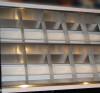### Summary measurement data

parameter meas. result remark
Color temperature 5158 K Natural white
Luminous intensity Iv 1192 Cd
Beam angle 89 deg
Power P 90 W
Power Factor 0.95 For every 1 kWh net power consumed, there has been 0.3 kVAhr for reactive power.
Luminous flux 2243 lm
Luminous efficacy 25 lm/W
CRI_Ra 97 Color Rendering Index.
Coordinates chromaticity diagram x=0.3417 and y=0.3539
Fitting 230V
L x W x H external dimensions 1240 x 295 x 90 mm External dimensions of the armature.
L x W luminous area 1170 x 246 mm Dimensions of the luminous area (used in Eulumdat file). This is the surface area of the open end of the luminaire.
form factor panel
General remarks The ambient temperature during the whole set of measurements was 24-27 deg C.Warm up effect: illuminance increases more than a factor of 2 during warming up. The warming up period is 3 minutes.

Voltage dependency: the illuminance and the consumed power depend linearly on the lamp voltage applied.

The Eulumdat file can be found via this link.

Measurement report (PDF) tbc

### Eulumdat light diagram

An interesting graph is the light diagram, indicating the intensity in the C0-C180 and the C90-C270 plane. This light diagram below comes from the program Qlumedit, that extracts these diagrams from an Eulumdat file.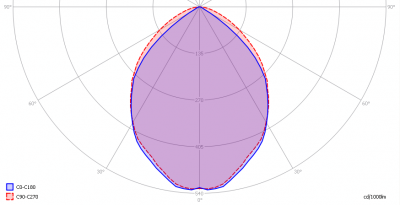The light diagram giving the radiation pattern.

It indicates the luminous intensity around the lamp. This light diagram is symmetrical to plane C0-C180 (perpendicular to length side of the lamp) and symmetrical to plane C90-C270 (alongside the length of the lamp).

The unit is Cd/1000lm, meaning the intensity in Cd assuming there would be 1000 lumen in the measured light bulb. This enables comparing different types of light bulbs and lamps.

### Illuminance Ev at 1 m distance, or luminous intensity Iv

Herewith the plot of the averaged luminous intensity Iv as a function of the inclination angle with the light bulb.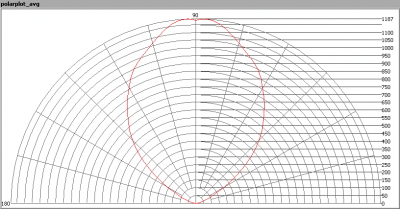The radiation pattern of the light bulb.

This radiation pattern is the average radiation, so per inclination angle the intensity results of all turn angles are averaged. This graph gives less information than does the Eulumdat light diagram graph. The results of averaging are needed to compute the lumen output.

This graph is not normalized to 1000 lm, but instead the intensity is directly given for this lamp.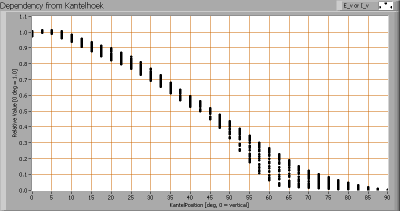Intensity data of every measured turn angle at each inclination angle.

This plot shows per inclination angle the intensity measurement results for each turn angle at that inclination angle. So at 45 degrees inclination angle, all the measurement results of illuminance for all measured turn angles are in the range of 45-53 % of the illuminance value at 0 degrees inclination angle.

When using the average values per inclination angle, the beam angle can be computed, being 89 degrees.

### Luminous flux

With the averaged illuminance data at 1 m distance, taken from the graph showing the averaged radiation pattern, it is possible to compute the luminous flux.

The result of this computation for this light spot is a luminous flux of 2243 lm.

### Luminous efficacy

The luminous flux being 2243 lm, and the power of the lightbulb being 90 W, yields a luminous efficacy of 25 lm/W.

A power factor of 0.95 means that for every 1 kWh net power consumed, a reactive component of 0.3 kVAr was needed.

 Light bulb voltage 230 V Light bulb current 412 mA Power P 90 W Apparent power S 95 VA Power factor 0.95

### Color temperature and Spectral power distribution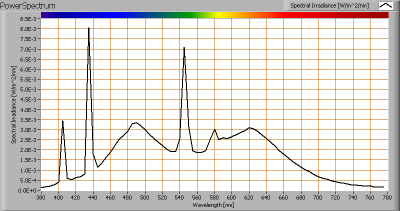The spectral power distribution of this light bulb.

The measured color temperature is about 5158 K which is natural white. The spectrum does not show any empty wavelengths, which means that this spectrum is most likely capable of rendering all colors.

This color temperature is measured straight underneath the light bulb. Below a graph showing the color temperature for different inclination angles.Color temperature as a function of inclination angle.

The maximum value of inclination angle is 80 degrees. Beyond that value there is virtually no intensity anymore. The color temperature decreases under increasing inclination angle, with max 3 %.

### Chromaticity diagram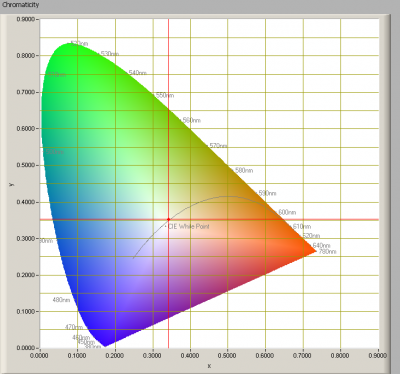The chromaticity space and the position of the lamp’s color coordinates in it.

The light coming from this lamp on the Planckian Locus (the black path in the graph).

Its coordinates are x=0.3417 and y=0.3539.

### Color Rendering Index (CRI) or also Ra

Herewith the image showing the CRI as well as how well different colors are represented (rendered). The higher the number, the better the resemblance with the color when a black body radiator would have been used (the sun, or an incandescent lamp).

Each color has an index Rx, and the first 8 indexes (R1 .. R8) are averaged to compute the Ra which is equivalent to the CRI.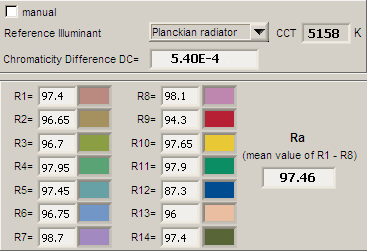CRI of the light of this lightbulb.

The value of 97 is higher than 80 which is considered a minimum value for indoor usage. Looking even at the values for R1 to R8, these are all very high meaning that these colors are rendered very well. Even the colors R9 – R14 have high values (these colors are not taken into account for this CRI calculation).

Note: the chromaticity difference is 0.0005 indicates the distance to the Planckian Locus. Its value is lower than 0.0054, which means that the calculated CRI result is meaningful.

### Voltage dependency

The dependency of a number of lamp parameters on the lamp voltage is determined. For this, the lamp voltage has been varied and its effect on the following lamp parameters measured: illuminance E_v [lx], color temperature CT or correlated color temperature CCT [K], the lamppower P [W] and the luminous efficacy [lm/W].Lamp voltage dependencies of certain light bulb parameters, where the value at 230 V is taken as 100 %.

The consumed power and the illuminance are dependent from lamp voltage variation, and their dependence is linear.

When the lamp voltage varies between 230 + and – 5 V, the illuminance varies less than 2.5 %. So sudden voltage variations in the lamp voltage will not be visible.

### Warm up effects

After switch on of a cold lamp, the effect of heating up of the lamp is measured on illuminance E_v [lx], color temperature CT or correlated color temperature CCT [K], the lamppower P [W] and the luminous efficacy [lm/W].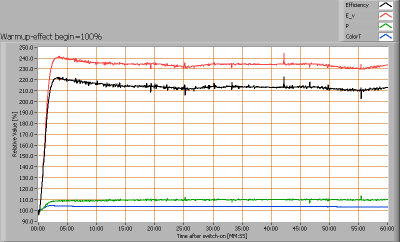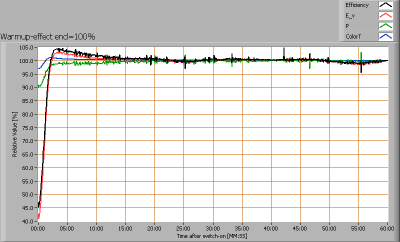Effect of warming up on different light bulb parameters. At top the 100 % level is put at begin, and at bottom at the end.

A more than two fold increase in illuminance due to the warming up of the lamp, which takes about 3 minutes.

The temperature variations of the ambient (from 24 to 27 deg) do have little effect (max 1 %), see also the second graph at the time period 10:00 – 60:00.